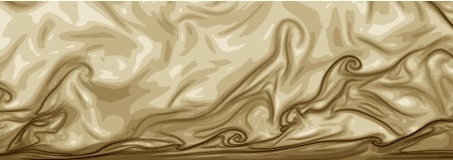efluidsSearch eFluids Search WWWAbout eFluids eFluids Education Focus   eFluids Books Tutorials Education Tools and Materials   Gallery of Videos Gallery of Images Gallery of Experiments Gallery of Problems Calculators and Applets   Bicycle Aerodynamics## Fluid Flow Calculators and Applets

Interactive Tools by M. van Rooij and H. Higuchi
• Unit Conversion
• Reynolds Number Calculator
• Mach Number Calculator
• Standard Atmosphere
• Sports Aerodynamics (spin, baseball and golf ball)
• Superposition of Elementary Flows Calculator
• Airfoil Analysis Applet
• Simulation of a Pendulum in a Fluid Calculator
• Sound Wave Propagation
• Shock Wave Simulation
• Interactive Tools at FluidMech.net
• Friction Factor Calculator - Virtual Moody Diagram.
• Bluff Body Drag Calculators which provide the drag and drag coefficient for an assortment of bluff bodies
• Drag vs Reynolds Number for cylinders and spheres
• Incompressible, Flat-Plate Boundary Layers using Re-1/5scalings
• Incompressible, Flat-Plate Boundary Layers using Re-1/7scalings
• Gravity-Capillary Waves Calculator
• Calculator for Waves in Solids
• Liquid Viscosity Calculator
• Liquid Thermal Conductivity Calculator
• Gas Property Calculator
• Interactive Tools by David Hill
• Systems of Units
• Operation of a Manometer
• Hydrostatic Forces on Plane Surfaces
• Dimensional Analysis
• Similitude
• Basic Pipe Flow Calculations
• Pipes in Parallel
• Interactive Tools by C. Wassgren, C.M. Krousgrill and P. Carmody
• Isentropic Flow Calculator
• Fanno Flow Calculator
• Rayleigh Flow Calculator
• Normal Shock Relations Calculator
• Oblique Shock Relations Calculator
• Expansion Fan Calculator
• Interactive Tools by Subrata Bhattacharjee.
• Unit Converter
• Tables and Charts
• Gas Dynamics: High Speed Flows of Perfect Gases
• Many, many thermodynamic calculators
• Interactive Tools by Alina A. Alexeenko
• Gas Dynamics Toolbox Applet
• Interactive Tools by Gossman Consulting, Inc.
• Flow calculator for pipes to determine if flow is turbulent
• Interactive Tools by Ferdi Hellweger
• UTFLOW - This applet computes and visualizes the EGL and HGL for a simple
linear pipe system
• Interactive Tools by Mark Mitchell
• Animated Demonstration of Bernoulli's Principle
• PAGE 1 | PAGE 2© 2013 iCentral | Editors | Fair Use | Contact Us | Banner image: Melissa Green | Last update: December 16, 2013